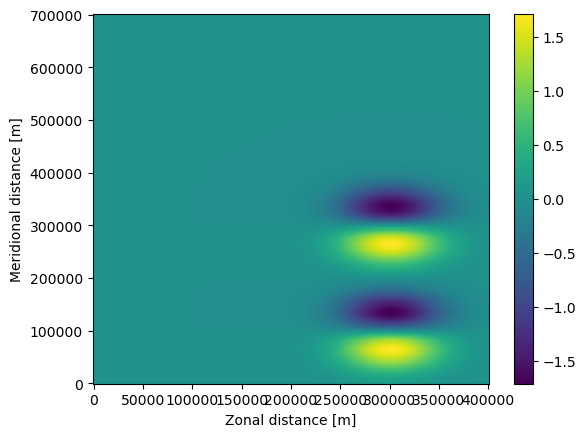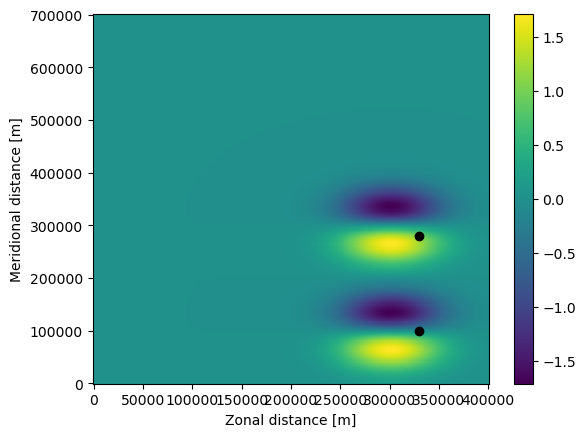# Quickstart to Parcels#

Welcome to a quick tutorial on Parcels. This is meant to get you started with the code, and give you a flavour of some of the key features of Parcels.

In this tutorial, we will first cover how to run a set of particles from a very simple idealised field. We will show how easy it is to run particles in time-backward mode. Then, we will show how to add custom behaviour to the particles. Then we will show how to run particles in a set of NetCDF files from external data. Then we will show how to use particles to sample a field such as temperature or sea surface height. And finally, we will show how to write a kernel that tracks the distance travelled by the particles.

Let’s start with importing the relevant modules. The key ones are all in the parcels package.

:

import math
from datetime import timedelta
from operator import attrgetter

import matplotlib.pyplot as plt
import numpy as np
import trajan as ta
import xarray as xr
from IPython.display import HTML
from matplotlib.animation import FuncAnimation

from parcels import (
FieldSet,
JITParticle,
ParticleSet,
Variable,
)


## Running particles in an idealised field#

The first step to running particles with Parcels is to define a FieldSet object, which is simply a collection of hydrodynamic fields. In this first case, we use a simple flow of two idealised moving eddies. That field can be downloaded using the download_example_dataset() function that comes with Parcels. Since we know that the files are in what’s called Parcels FieldSet format, we can call these files using the function FieldSet.from_parcels().

:

example_dataset_folder = download_example_dataset("MovingEddies_data")

fieldset = FieldSet.from_parcels(f"{example_dataset_folder}/moving_eddies")


The fieldset can then be visualised with e.g. matplotlib.pyplot.pcolormesh(). To show the zonal velocity (U), give the commands below. Note that we first have to load the fieldset with fieldset.computeTimeChunk(time=0, dt=1) to load the first time frame of the fieldset.

:

fieldset.computeTimeChunk(time=0, dt=1)

plt.pcolormesh(fieldset.U.grid.lon, fieldset.U.grid.lat, fieldset.U.data[0, :, :])
plt.xlabel("Zonal distance [m]")
plt.ylabel("Meridional distance [m]")
plt.colorbar()
plt.show()The next step is to define a ParticleSet. In this case, we start 2 particles at locations (330km, 100km) and (330km, 280km) using the from_list constructor method, that are advected on the fieldset we defined above. Note that we use JITParticle as pclass, because we will be executing the advection in JIT (Just-In-Time) mode. The alternative is to run in scipy mode, in which case pclass is ScipyParticle

:

pset = ParticleSet.from_list(
fieldset=fieldset,  # the fields on which the particles are advected
pclass=JITParticle,  # the type of particles (JITParticle or ScipyParticle)
lon=[3.3e5, 3.3e5],  # a vector of release longitudes
lat=[1e5, 2.8e5],  # a vector of release latitudes
)


Print the ParticleSet to see where they start

:

print(pset)

P(lon=330000.000000, lat=100000.000000, depth=0.000000, time=not_yet_set)
P(lon=330000.000000, lat=280000.000000, depth=0.000000, time=not_yet_set)


This output shows for each particle the (longitude, latitude, depth, time). Note that in this case the time is not_yet_set, that is because we didn’t specify a time when we defined the pset.

To plot the positions of these particles on the zonal velocity, use the following command

:

plt.pcolormesh(fieldset.U.grid.lon, fieldset.U.grid.lat, fieldset.U.data[0, :, :])
plt.xlabel("Zonal distance [m]")
plt.ylabel("Meridional distance [m]")
plt.colorbar()

plt.plot(pset.lon, pset.lat, "ko")
plt.show()The final step is to run (or ‘execute’) the ParticelSet. We run the particles using the AdvectionRK4 kernel, which is a 4th order Runge-Kutte implementation that comes with Parcels. We run the particles for 6 days (using the timedelta function from datetime), at an RK4 timestep of 5 minutes. We store the trajectory information at an interval of 1 hour in a file called EddyParticles.zarr. Because time was not_yet_set, the particles will be advected from the first date available in the fieldset, which is the default behaviour.

:

output_file = pset.ParticleFile(
name="EddyParticles.zarr",  # the file name
outputdt=timedelta(hours=1),  # the time step of the outputs
)
pset.execute(
AdvectionRK4,  # the kernel (which defines how particles move)
runtime=timedelta(days=6),  # the total length of the run
dt=timedelta(minutes=5),  # the timestep of the kernel
output_file=output_file,
)

INFO: Compiled ArrayJITParticleAdvectionRK4 ==> /var/folders/x0/1qxj679n55zcybylvdsb4bxh0000gq/T/parcels-503/a0bf81d0bd8a7a1d958e92931c40923e_0.c


The code should have run, which can be confirmed by printing and plotting the ParticleSet again

:

print(pset)

plt.pcolormesh(fieldset.U.grid.lon, fieldset.U.grid.lat, fieldset.U.data[0, :, :])
plt.xlabel("Zonal distance [m]")
plt.ylabel("Meridional distance [m]")
plt.colorbar()

plt.plot(pset.lon, pset.lat, "ko")
plt.show()

P(lon=227200.015625, lat=82139.953125, depth=0.000000, time=518400.000000)
P(lon=261314.703125, lat=320586.687500, depth=0.000000, time=518400.000000)Note that both the particles (the black dots) and the U field have moved in the plot above. Also, the time of the particles is now 518400 seconds, which is 6 days.

The trajectories in the EddyParticles.zarr file can be quickly plotted using xr.open_zarr() (note that the lon and lat arrays need to be transposed with .T).

:

ds = xr.open_zarr("EddyParticles.zarr")

plt.plot(ds.lon.T, ds.lat.T, ".-")
plt.xlabel("Zonal distance [m]")
plt.ylabel("Meridional distance [m]")
plt.show()Using the FuncAnimation() method, we can show the trajectories as an animation and watch the particles go!

:

%%capture
fig = plt.figure(figsize=(7, 5), constrained_layout=True)

ax.set_ylabel("Meridional distance [m]")
ax.set_xlabel("Zonal distance [m]")
ax.set_xlim(0, 4e5)
ax.set_ylim(0, 7e5)

# show only every fifth output (for speed in creating the animation)
timerange = np.unique(ds["time"].values)[::5]

# Indices of the data where time = 0
time_id = np.where(ds["time"] == timerange)

sc = ax.scatter(ds["lon"].values[time_id], ds["lat"].values[time_id])

t = str(timerange.astype("timedelta64[h]"))
title = ax.set_title(f"Particles at t = {t}")

def animate(i):
t = str(timerange[i].astype("timedelta64[h]"))
title.set_text(f"Particles at t = {t}")

time_id = np.where(ds["time"] == timerange[i])
sc.set_offsets(np.c_[ds["lon"].values[time_id], ds["lat"].values[time_id]])

anim = FuncAnimation(fig, animate, frames=len(timerange), interval=100)

:

HTML(anim.to_jshtml())

:


## Running particles in backward time#

Running particles in backward time is extremely simple: just provide a dt < 0.

:

output_file = pset.ParticleFile(
name="EddyParticles_Bwd.zarr",  # the file name
outputdt=timedelta(hours=1),  # the time step of the outputs
)
pset.execute(
dt=-timedelta(minutes=5),  # negative timestep for backward run
runtime=timedelta(days=6),  # the run time
output_file=output_file,
)


Now print the particles again, and see that they (except for some round-off errors) returned to their original position

:

print(pset)

plt.pcolormesh(fieldset.U.grid.lon, fieldset.U.grid.lat, fieldset.U.data[0, :, :])
plt.xlabel("Zonal distance [m]")
plt.ylabel("Meridional distance [m]")
plt.colorbar()

plt.plot(pset.lon, pset.lat, "ko")
plt.show()

P(lon=329999.937500, lat=99999.492188, depth=0.000000, time=0.000000)
P(lon=329999.781250, lat=279999.750000, depth=0.000000, time=0.000000)## Adding a custom behaviour kernel#

A key feature of Parcels is the ability to quickly create very simple kernels, and add them to the execution. Kernels are little snippets of code that are run during exection of the particles.

In this example, we’ll create a simple kernel where particles obtain an extra 2 m/s westward velocity after 1 day. Of course, this is not very realistic scenario, but it nicely illustrates the power of custom kernels.

:

def WestVel(particle, fieldset, time):
if time > 86400:
uvel = -2.0
particle.lon += uvel * particle.dt


Now reset the ParticleSet again, and re-execute. Note that we have now changed the first argument of pset.execute() to be a list of [AdvectionRK4, WestVel].

:

pset = ParticleSet.from_list(
fieldset=fieldset, pclass=JITParticle, lon=[3.3e5, 3.3e5], lat=[1e5, 2.8e5]
)

output_file = pset.ParticleFile(
name="EddyParticles_WestVel.zarr", outputdt=timedelta(hours=1)
)
pset.execute(
[AdvectionRK4, WestVel],  # simply combine the Kernels in a list
runtime=timedelta(days=2),
dt=timedelta(minutes=5),
output_file=output_file,
)

INFO: Compiled ArrayJITParticleAdvectionRK4WestVel ==> /var/folders/x0/1qxj679n55zcybylvdsb4bxh0000gq/T/parcels-503/83147522a881619ac230e507e4b08c01_0.c


And now plot this new trajectory file

:

ds = xr.open_zarr("EddyParticles_WestVel.zarr")

plt.plot(ds.lon.T, ds.lat.T, ".-")
plt.xlabel("Zonal distance [m]")
plt.ylabel("Meridional distance [m]")
plt.show()## Reading in data from arbritrary NetCDF files#

In most cases, you will want to advect particles within pre-computed velocity fields. If these velocity fields are stored in NetCDF format, it is fairly easy to load them into the FieldSet.from_netcdf() function.

The examples directory contains a set of GlobCurrent files of the region around South Africa.

First, define the names of the files containing the zonal (U) and meridional (V) velocities. You can use wildcards (*) and the filenames for U and V can be the same (as in this case)

: